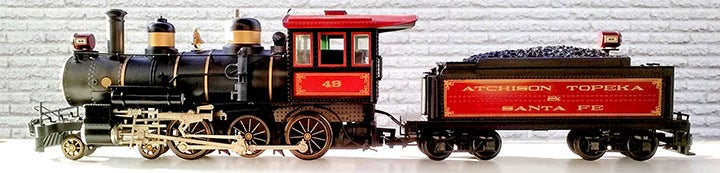1 - 9 of 9 Posts

#### John J

·
Joined
·
5,334 Posts
Discussion Starter · ·
Ok  when one is talking about switch tracks and  they say it is a   # 6.    Does it mean that the  larger the number  the less the curve of the switch?

Or  the  higher the number the  tighter the curve of the switch?

Any one got a chart to covert   numbers to  diamaters?

When it comes to Scale I have burned into my brain    THE SMALLER THE NUMBER THE LARGER THE OBJECT.#### GarryNCGRR

·
##### Registered
Joined
·
100 Posts
Hi JJ......the larger the number of the switch,  the wider the curve.  A number 4 switch is tighter than a number 6 and so on.
Hope this helps.
Garry  NCGRR

#### rkapuaala

·
Joined
·
2,007 Posts
Whats the radius on a number 6 and on a number 4?

#### CCSII

·
##### Registered
Joined
·
350 Posts
Actually, I think numbered switches don't have a "radius."

Radius switches diverge from the straight through track on a curve that has a radius (i.e. Aristo Wide Radius switch is a 10' diameter - 5' radius curve.)

Numbered switches diverge from the switch at an angle. The number of the switch is the ratio of the number of units the diverging track goes forward for a one unit distance from the through track. In other words a number 4 switch would have a diverging track that separated from the through track by 1 inch for every four inches of length on the diverging track. On a number 8 switch, the diverging track would be 8 inches long before it had diverged 1 inch.

#### twolfe

·
##### Registered
Joined
·
6 Posts
I'm using #6's combined with 20' D which appear(??) to work fine, albeit lots of back yard space is required.

"T"

#### blackburn49

·
Joined
·
3,315 Posts
Posted By CCSII on 03/02/2008 8:51 PM

Numbered switches diverge from the switch at an angle. The number of the switch is the ratio of the number of units the diverging track goes forward for a one unit distance from the through track. In other words a number 4 switch would have a diverging track that separated from the through track by 1 inch for every four inches of length on the diverging track. On a number 8 switch, the diverging track would be 8 inches long before it had diverged 1 inch.
Finally, an explanation of the numbers that makes sense. Thanks.

#### Schlosser

·
Joined
·
113 Posts
For numbered switches, there is no right angle involved. The number refers to what the mathematicians call an Isosceles triangle, a triangle with two equal sides. For switches, the two sides (of the triangle) are 4 or some number and the base is always 1. If you measure the '1' straight up or down to the diverging rail, you're making the number of the switch larger than you had wanted.

To belabor the point, a Number 1 switch forms an equilateral triangle, all sides the same length with a frog angle of 60 degrees. But if you measure out 1 distance and then measure straight up the same distance, that will form a 45 degree right triangle; the frog will be 45 degrees and would be a Number 1.4 switch.

Art

#### CCSII

·
##### Registered
Joined
·
350 Posts
True

For practical purposes though, in switch numbers which are normally used, the right triangle is close enough in approximation to the isosceles.

·